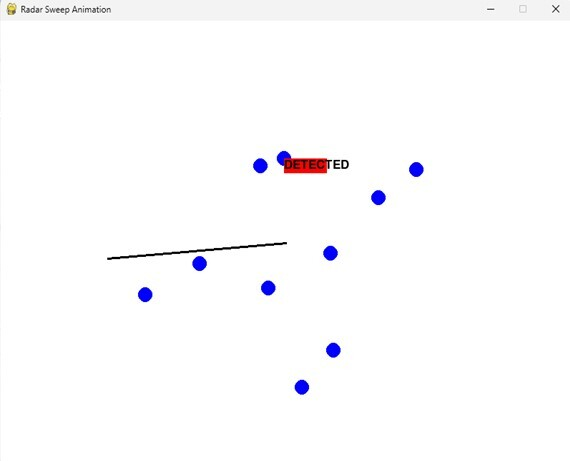# Creating a radar sweep animation using Pygame in Python

Pygame is a cross-platform set of Python modules designed for writing video games. It includes computer graphics and sound libraries designed to be used with the Python programming language. Pygame is not a game development engine, but rather a set of tools and libraries that allow developers to create 2D games in Python.

Pygame provides a variety of functions and classes to help developers create games, including image loading and manipulation, sound playback and recording, keyboard and mouse input handling, sprite and group management, and collision detection. It also includes built-in support for common game development tasks such as animation, scrolling, and tile-based maps.

Pygame is open source and free to use, and it runs on Windows, macOS, Linux, and other platforms. It is often used in educational settings as an introduction to game development or as a tool for teaching programming concepts.

## Components of a Radar Sweep Animation

A basic radar sweep animation consists of the following components −

• Radar Circle − This is the circle that represents the range of the radar. It is centered at the origin and has a fixed radius.

• Radar Sweep − This is a line that rotates around the center of the circle. It represents the beam emitting from the radar and sweeps across 360 degrees.

• Radar Targets − These are the objects that we want to detect using the radar. They are represented as points on the screen.

Now that we know the components of a radar sweep animation, let's dive into the implementation using Pygame.

## Prerequisites

Before we dive into the task few things should is expected to be installed onto your

system −

List of recommended settings −

• pip install Numpy, pygame and Math

• It is expected that the user will have access to any standalone IDE such as VSCode, PyCharm, Atom or Sublime text.

• Even online Python compilers can also be used such as Kaggle.com, Google Cloud platform or any other will do.

• Updated version of Python. At the time of writing the article I have used 3.10.9 version.

• Knowledge of the use of Jupyter notebook.

• Knowledge and application of virtual environment would be beneficial but not required.

• It is also expected that the person will have a good understanding of statistics and mathematics.

## Implementation Details

Importing Libraries − We will first import the necessary libraries - Pygame, NumPy and Math.

import pygame
import math
import random


Initializing the Game Window − We will initialize the game window with the required width and height using the Pygame library.

WIDTH = 800
HEIGHT = 600
pygame.init()
screen = pygame.display.set_mode((WIDTH, HEIGHT))
clock = pygame.time.Clock()


Setting up the Game Environment − We will set up the game environment by defining the colors, background, and frame rate of the animation.

WHITE = (255, 255, 255)
BLACK = (0, 0, 0)
RED = (255, 0, 0)
BLUE = (0, 0, 255)


### Set the frame rate of the animation

Defining the Radar Circle − We will define the radar circle with the required radius and center coordinates. We will also set the color and line thickness of the circle.

radar_circle_radius = 200


Defining the Radar Sweep − We will define the radar sweep by setting the initial angle to zero degrees and incrementing it in each frame to make it sweep across 360 degrees. We will also set the color and thickness of the sweep line.

radar_sweep_angle = 0
def update():
# Increment the angle in each frame
# Draw the radar sweep line


Defining the Radar Targets − We will define the radar targets using random x and y coordinates within the range of the radar circle. We will also set the color and radius of the targets.

num_targets = 10
targets = []
for i in range(num_targets):
targets.append((x, y))
for target in targets:
distance_to_target = math.sqrt((target - x) ** 2 + (target - y) ** 2)
pygame.draw.rect(screen, RED, (target, target, 60, 20))
font = pygame.font.SysFont(None, 25)
text = font.render("DETECTED", True, BLACK)
screen.blit(text, (target, target))


Running the Game − We will run the game by creating a pygame window, setting the necessary event handlers and running the game loop.

# Main game loop
running = True
while running:
for event in pygame.event.get():
if event.type == pygame.QUIT:
running = False
screen.fill(WHITE)
update()
pygame.display.update()
clock.tick(FPS)
# Quit the game
pygame.quit()


## Final Program, code

import pygame
import math
import random
# Initializing the Game Window:
WIDTH = 800
HEIGHT = 600
pygame.init()
screen = pygame.display.set_mode((WIDTH, HEIGHT))
clock = pygame.time.Clock()
# Defining colors:
WHITE = (255, 255, 255)
BLACK = (0, 0, 0)
RED = (255, 0, 0)
BLUE = (0, 0, 255)
# Set the frame rate of the animation
FPS = 60
num_targets = 10
targets = []
for i in range(num_targets):
targets.append((x, y))
def update():
# Increment the angle in each frame
# Draw the radar sweep line
for target in targets:
distance_to_target = math.sqrt((target - x) ** 2 + (target - y)** 2)
pygame.draw.rect(screen, RED, (target, target, 60, 20))
font = pygame.font.SysFont(None, 25)
text = font.render("DETECTED", True, BLACK)
screen.blit(text, (target, target))
# Main game loop
running = True
while running:
for event in pygame.event.get():
if event.type == pygame.QUIT:
running = False
screen.fill(WHITE)
update()
pygame.display.update()
clock.tick(FPS)
# Quit the game
pygame.quit()We can see the output of the program where the animation of the game can be observed.

## Conclusion

In this document, we explored how to create a radar sweep animation using Pygame in Python. We learned about the components of a radar sweep animation and walked through the implementation details using code snippets and real-world examples. Pygame provides a user-friendly API for game development and is a great choice for creating 2D video games and animations. With the knowledge gained from this document, you should now be able to create your own radar sweep animations using Pygame.

Updated on: 25-Apr-2023

314 Views